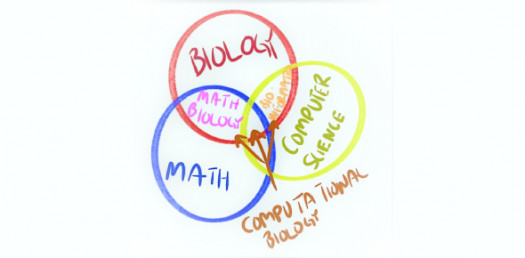# Basic Aptitude Test On Math And Biology! Quiz

5 Questions | Total Attempts: 108SettingsThere is a lot that we have had to learn in math and biology class and below is a basic aptitude test on math and biology that is designed to see just how much revision you may need to do before the final exams. Do give it a try and keep an eye out for other tests just like it.

Questions and Answers
• 1.
Solve for n: 4n = 28
• A.

32

• B.

24

• C.

7

• D.

6

• 2.
Solve if:   a = 4, b = 9, c = 5 a + b - c = _____
• A.

6

• B.

18

• C.

17

• D.

8

• 3.
Solve if:   a = 4, b = 9, c = 5 3a + 7 = _____
• A.

19

• B.

21

• C.

20 13

• D.

14

• 4.
There are ___________ different types of joints (how many?)
• 5.
The different joints in our body are: (check all that are correct)
• A.

Doorknob

• B.

Hinge

• C.

Immovable

• D.

Swinging

• E.

Pivot

• F.

Ball and Socket

• G.

Ribs

Related Topics